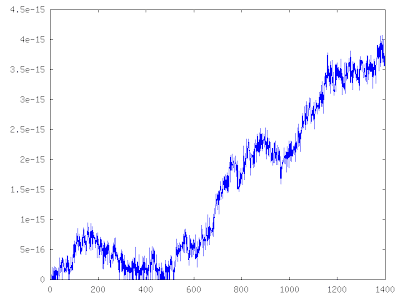## Friday, January 9, 2009

### Fibonacci Numbers

Gilbert Strang has an interesting example in one of his linear algebra lectures on eigenvalues that is relevant to the articles I've written on speeding up calculations in Octave.

You might be familiar with the Fibonacci numbers, they are calculated by a two term recurrence:We've already seen that recasting a calculation in terms of a matrix vector multiply or matrix vector solve is an important skill for writing good fast code in Octave. Strang demonstrates this at about halfway through the above lecture. The first order system that describes the generation of Fibonacci numbers is:The nth term of the sequence is calculated by taking the nth power of A and applying it to the initial conditions:When you diagonalize a matrix the powers of the matrix become very easy to calculate:If you aren't familiar with the concept of eigenvalues and eigenvectors and diagonalizing a matrix I would highly recommend the linear algebra lectures on MIT's OCW website.

Here's the Octave code to accomplish the above. If you want, you can use tic() and toc() for timing. On my laptop the matrix method is about three times faster than the for loop method. What kind of results do you get? This advantage will grow as you go further into the sequence because the amount of work stays constant for the matrix method, but grows linearly for the recurrence method.

%% Our 'fibonacci' operator:
A = [1,1;1,0]
%% our initial conditions:
u_0 = [0;1];

%% get the matrix of eignevectors, V, and the matrix of eigenvalues, lambda
[V,lambda] = eig(A)

%% A = V lambda V^{-1}

Vinv = inverse(V)

%% This gives us an idea of the error introduced by calculating the
%% inverse with finite precision arithmetic:
Error = A - V*lambda*Vinv

n = 1400;
F = zeros(n,2);
%% Find the 100th Fibonaci number:
F(100,:) = V * (lambda^100) * Vinv * u_0;

%% check our answer with a simple loop:
fibo = zeros(n,1);
fibo(1) = 0;
fibo(2) = 1;
for i=3:n
fibo(i) = fibo(i-1) + fibo(i-2);
endfor

for i=2:n
F(i,:) = V * (lambda^i) * Vinv *u_0;
endfor

relative_error = abs( ( F(3:n,2) - fibo(3:n) ) ./ fibo(3:n) );

One thing to note is that one of the eigenvalues of our matrix is greater than one. This has implications for the numerical stability of the above method for calculating terms in the Fibonacci sequence. It means that the initial tiny round-off error in our eigenvector matrix inverse will be amplified as we continue to calculate higher and higher terms in the sequence. This behaviour is shown in the plot below, luckily the error starts out very small, and doesn't grow too large before we run out of range in the 32bit floating point numbers on the machine.What's the answer? The 100th Fibonacci number is approximately 2.1892e20.